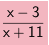Home > AC > Chapter 11 > Lesson 11.1.2 > Problem11-25

11-25.
1. Use your method for multiplying and dividing fractions to simplify the expressions below. Homework Help ✎

1.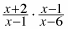2.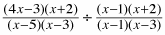3.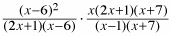4.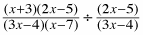5.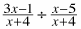6.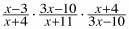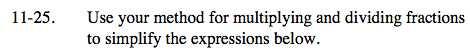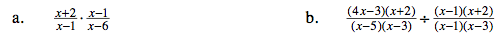Multiply and look for factors that make 1.

Dividing by a fraction is the
same as multiplying by its reciprocal.

Multiply the fractions and look for factors that make one.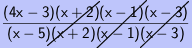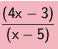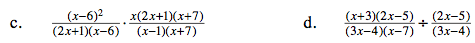See part (b).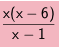See part (b).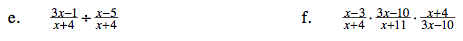See part (b).

See part (b).